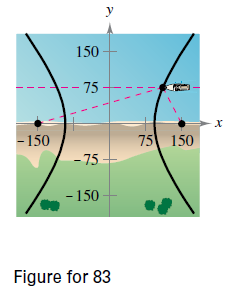Chapter 10.1, Problem 87E

Chapter
Section
Textbook Problem

# Navigation LORAN (long distance radio navigation) for aircraft and ships uses synchronized pulses transmitted by widely separated transmitting stations. These pulses travel at the speed of light (186,000 miles per second). The difference in the times of arrival of these pulses at an aircraft or ship is constant on a hyperbola having the transmitting stations as foci. Assume that two stations, 300 miles apart, are positioned on a rectangular coordinate system at (_150, 0) and (150, 0) and that a ship is traveling on a path with coordinates (x, 75) (see figure). Find the x-coordinate of the position of the ship when the time difference between the pulses from the transmitting stations is 1000 microseconds (0.001 second).To determine

To calculate: The x co-ordinate of the position of the ship when the difference of the time interval between the pulses of the transmitting stations is 0.001 seconds.

Explanation

Given:

The LARON pulses travel in a speed of 186,000 miles/second. The difference in the times of arrival of these pulses from the station is constant. The two stations 300 miles apart are on rectangular co-ordinates (-150, 0) and (150, 0) and the ship is travelling on the point (x, 75)

Formula Used:

The standard equation of hyperbola,

x2a2y2b2=1

Calculation:

The pulses travel in a speed of 186,000 miles per second. That means, in a second they travel 186,000 miles. Therefore, in a microsecond (0.001 second) they travel 186 miles. As the difference between the time of arrival of these pulses from the stations is constant which in this case is 0.001 seconds it had already moved 186 miles by then. Therefore, it satisfies the difference between the points and is equal to 186 miles.

According to the problem:

2c=300 mile

c=150

and

2a=186000×0

### Still sussing out bartleby?

Check out a sample textbook solution.

See a sample solution

#### The Solution to Your Study Problems

Bartleby provides explanations to thousands of textbook problems written by our experts, many with advanced degrees!

Get Started

#### Solve the equations in Exercises 126. 2(x21)x2+1x4x2+1x2+1=0

Finite Mathematics and Applied Calculus (MindTap Course List)

#### Place the following set of n = 20 scores in a frequency distribution table.

Essentials of Statistics for The Behavioral Sciences (MindTap Course List)

#### In problems 23-58, perform the indicated operations and simplify. (x3+x1)(x+2)

Mathematical Applications for the Management, Life, and Social Sciences